Скачать презентацию COMPUTATIONAL ASPECTS OF LOCAL REGRESSION MODELLING taking spatial

106fa50b61ea6318fe5718bb9eec7922.ppt

• Количество слайдов: 52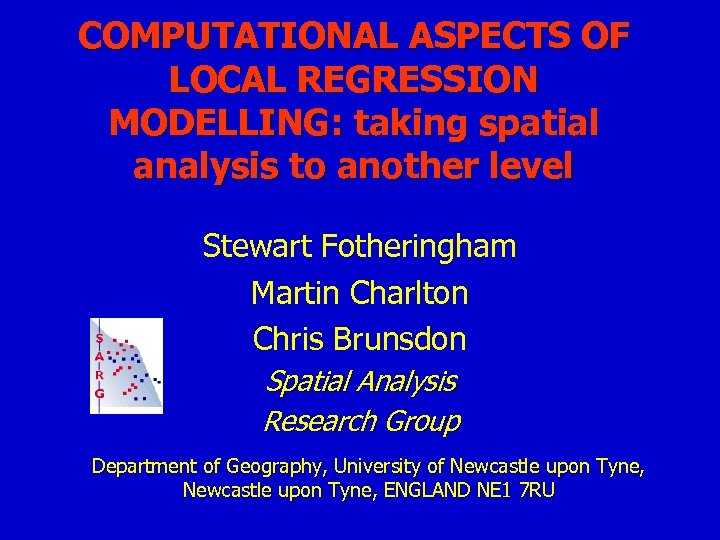COMPUTATIONAL ASPECTS OF LOCAL REGRESSION MODELLING: taking spatial analysis to another level Stewart Fotheringham Martin Charlton Chris Brunsdon Spatial Analysis Research Group Department of Geography, University of Newcastle upon Tyne, ENGLAND NE 1 7 RU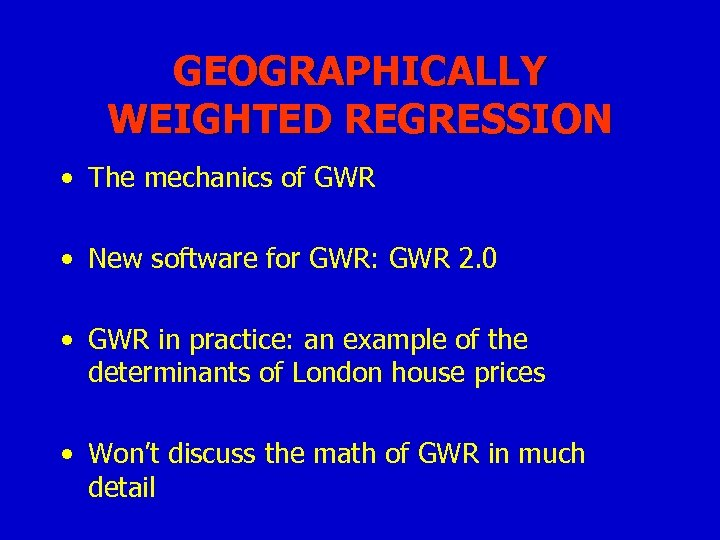GEOGRAPHICALLY WEIGHTED REGRESSION • The mechanics of GWR • New software for GWR: GWR 2. 0 • GWR in practice: an example of the determinants of London house prices • Won’t discuss the math of GWR in much detail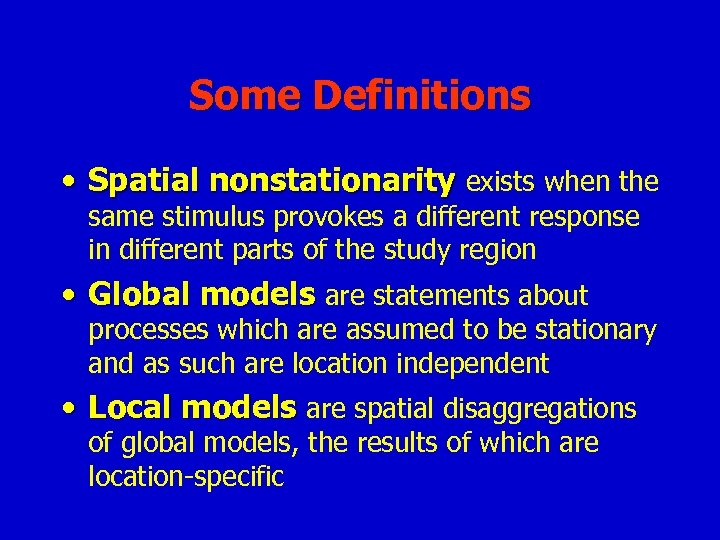Some Definitions • Spatial nonstationarity exists when the same stimulus provokes a different response in different parts of the study region • Global models are statements about processes which are assumed to be stationary and as such are location independent • Local models are spatial disaggregations of global models, the results of which are location-specific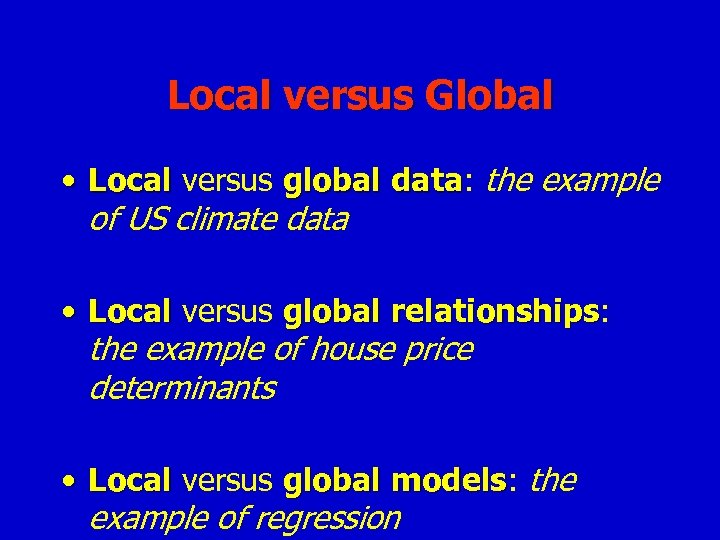Local versus Global • Local versus global data: the example data of US climate data • Local versus global relationships: relationships the example of house price determinants • Local versus global models: the models example of regression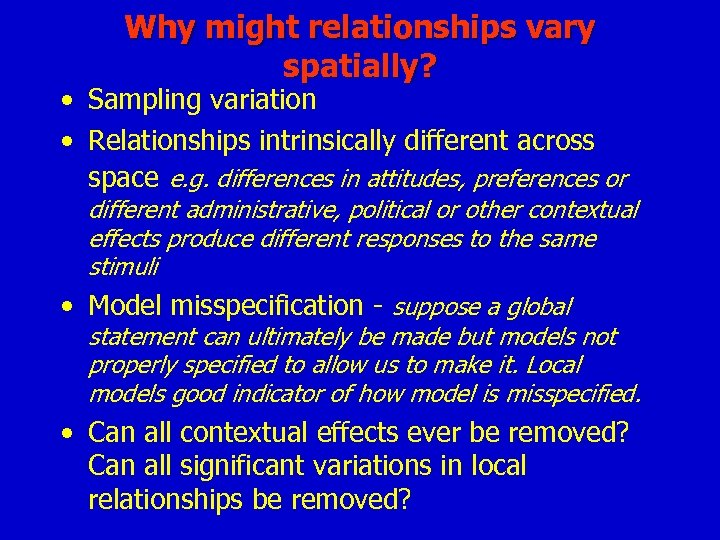Why might relationships vary spatially? • Sampling variation • Relationships intrinsically different across space e. g. differences in attitudes, preferences or different administrative, political or other contextual effects produce different responses to the same stimuli • Model misspecification - suppose a global statement can ultimately be made but models not properly specified to allow us to make it. Local models good indicator of how model is misspecified. • Can all contextual effects ever be removed? Can all significant variations in local relationships be removed?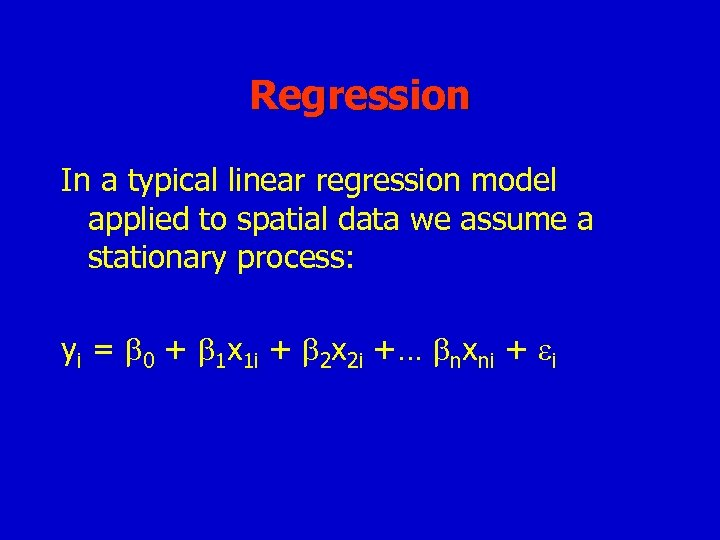Regression In a typical linear regression model applied to spatial data we assume a stationary process: yi = 0 + 1 x 1 i + 2 x 2 i +… nxni + i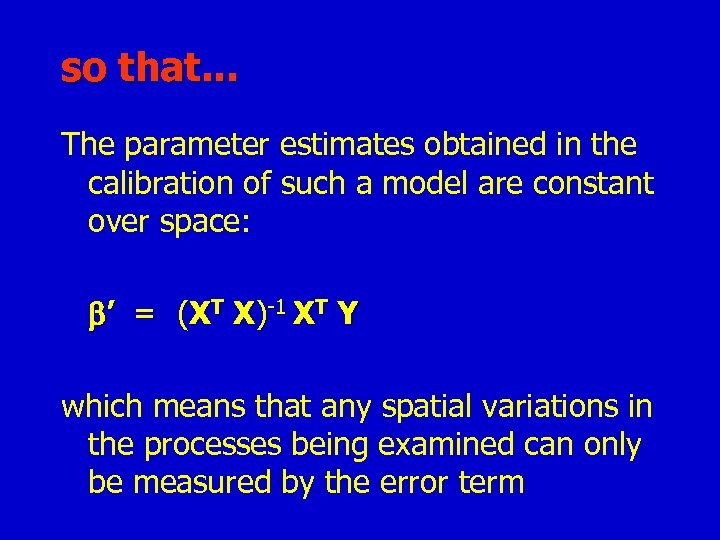so that. . . The parameter estimates obtained in the calibration of such a model are constant over space: ’ = (XT X)-1 XT Y which means that any spatial variations in the processes being examined can only be measured by the error term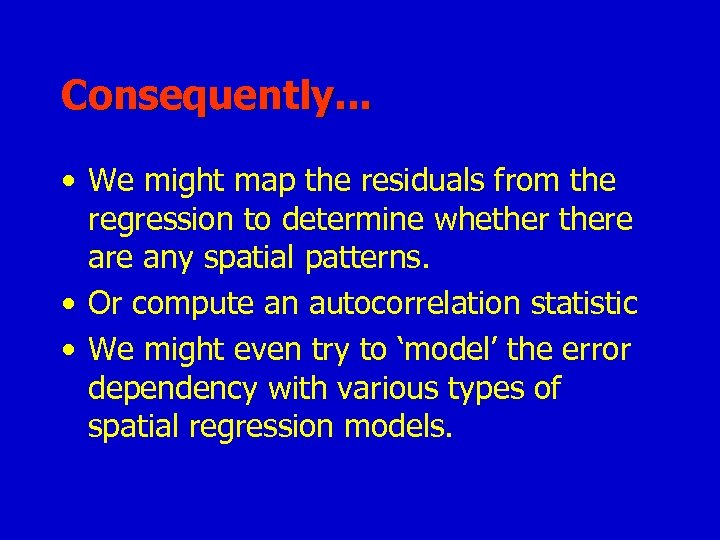Consequently. . . • We might map the residuals from the regression to determine whethere any spatial patterns. • Or compute an autocorrelation statistic • We might even try to ‘model’ the error dependency with various types of spatial regression models.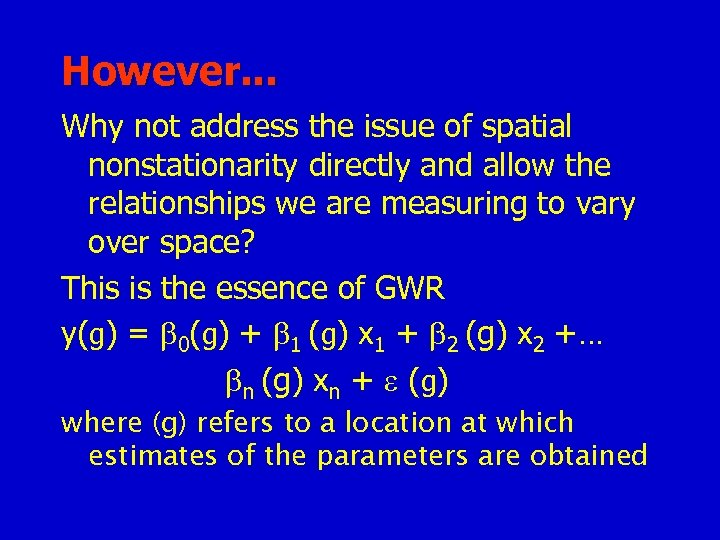However. . . Why not address the issue of spatial nonstationarity directly and allow the relationships we are measuring to vary over space? This is the essence of GWR y(g) = 0(g) + 1 (g) x 1 + 2 (g) x 2 +… n (g) xn + (g) where (g) refers to a location at which estimates of the parameters are obtained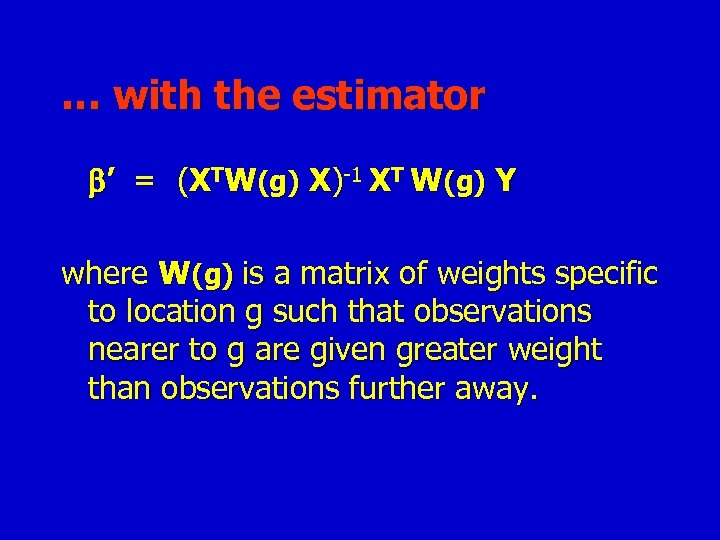… with the estimator ’ = (XTW(g) X)-1 XT W(g) Y where W(g) is a matrix of weights specific to location g such that observations nearer to g are given greater weight than observations further away.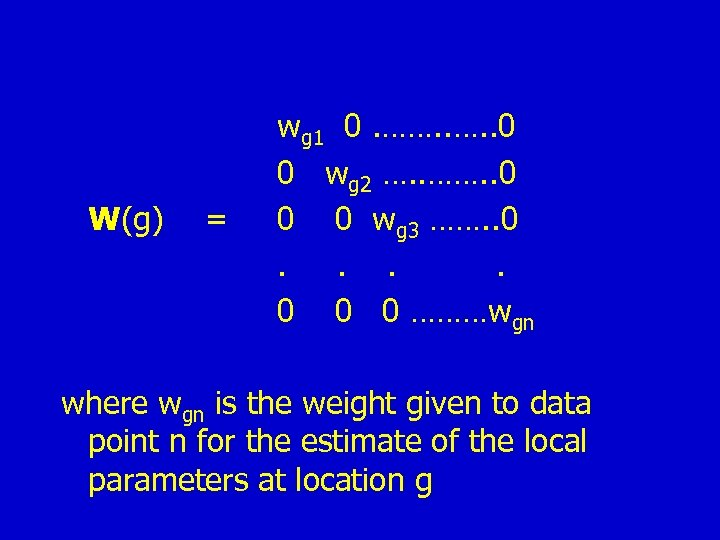W(g) = wg 1 0. ……. . 0 0 wg 2 …. . ……. . 0 0 0 wg 3 ……. . 0 0 0 ………wgn where wgn is the weight given to data point n for the estimate of the local parameters at location g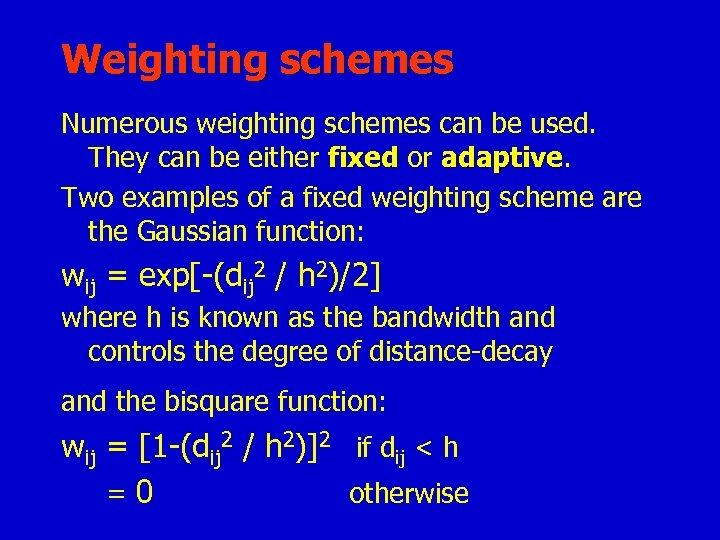Weighting schemes Numerous weighting schemes can be used. They can be either fixed or adaptive Two examples of a fixed weighting scheme are the Gaussian function: wij = exp[-(dij 2 / h 2)/2] where h is known as the bandwidth and controls the degree of distance-decay and the bisquare function: wij = [1 -(dij 2 / h 2)]2 if dij < h =0 otherwise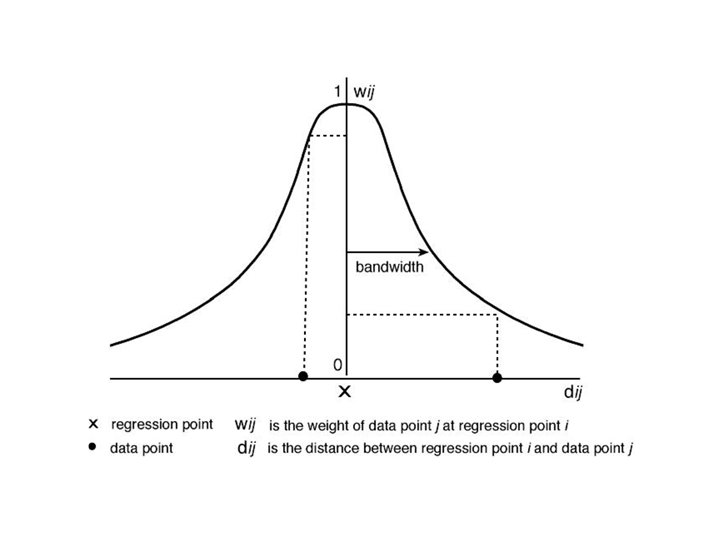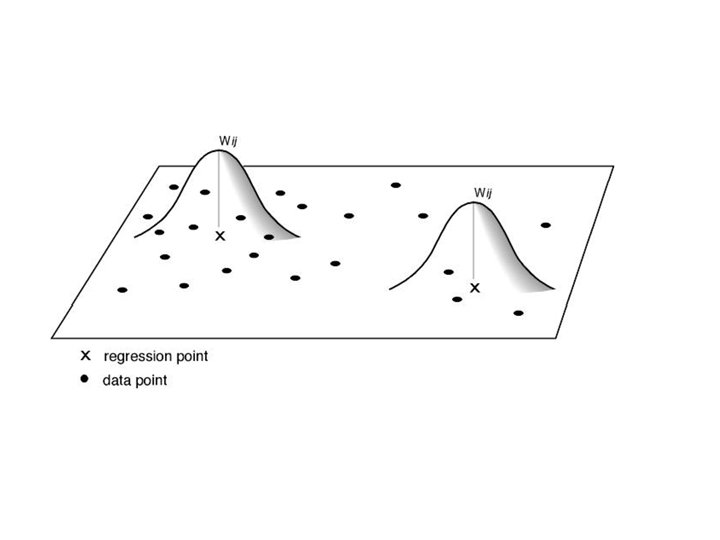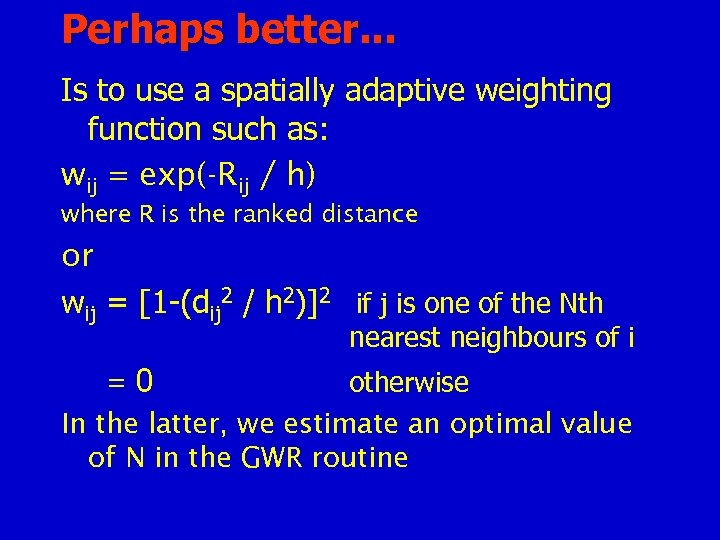Perhaps better. . . Is to use a spatially adaptive weighting function such as: wij = exp(-Rij / h) where R is the ranked distance or wij = [1 -(dij 2 / h 2)]2 if j is one of the Nth nearest neighbours of i =0 otherwise In the latter, we estimate an optimal value of N in the GWR routine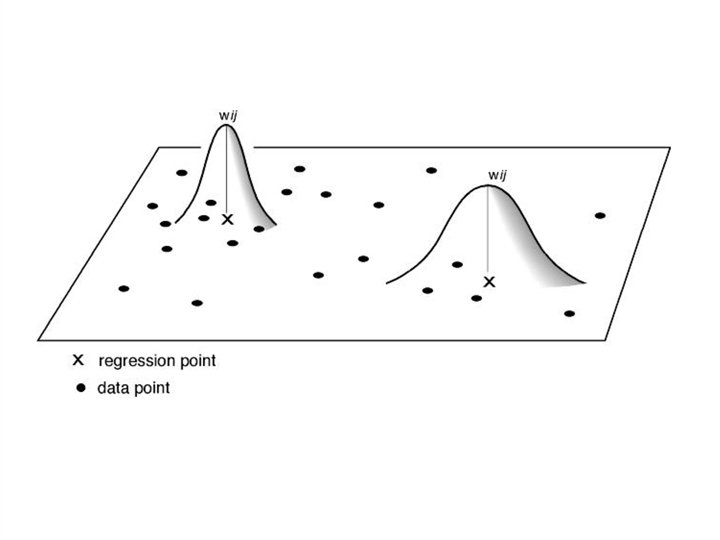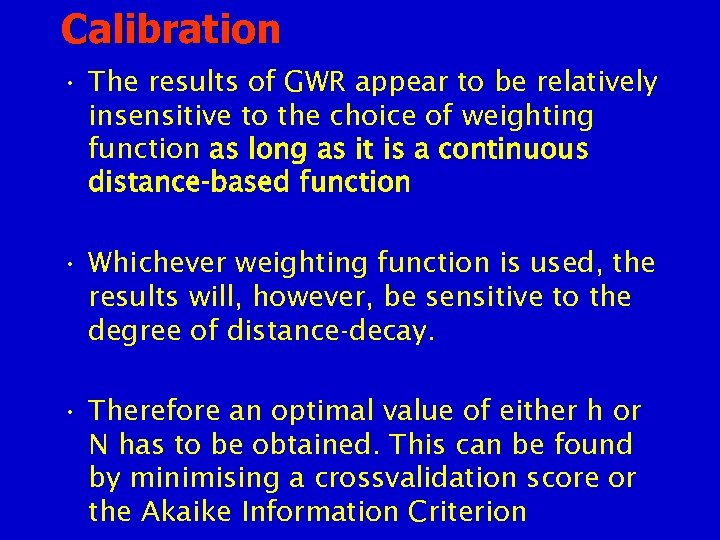Calibration • The results of GWR appear to be relatively insensitive to the choice of weighting function as long as it is a continuous distance-based function • Whichever weighting function is used, the results will, however, be sensitive to the degree of distance-decay. • Therefore an optimal value of either h or N has to be obtained. This can be found by minimising a crossvalidation score or the Akaike Information Criterion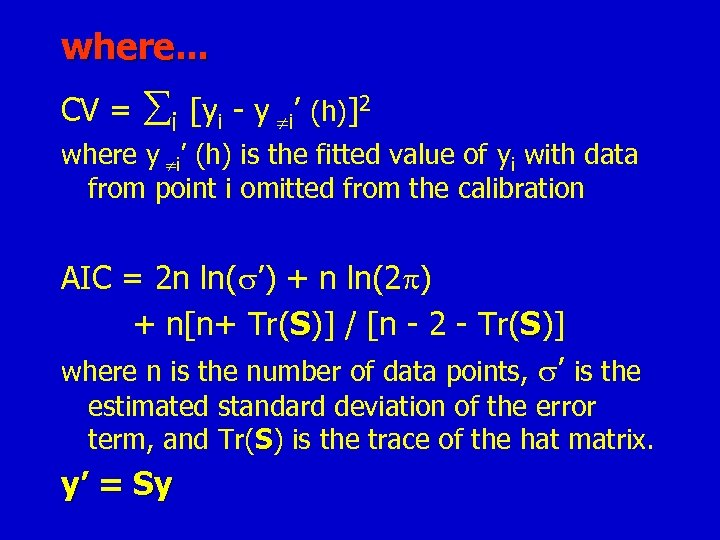where. . . CV = i [yi - y i’ (h)]2 where y i’ (h) is the fitted value of yi with data from point i omitted from the calibration AIC = 2 n ln( ’) + n ln(2 ) + n[n+ Tr(S)] / [n - 2 - Tr(S)] where n is the number of data points, ’ is the estimated standard deviation of the error term, and Tr(S) is the trace of the hat matrix. y’ = Sy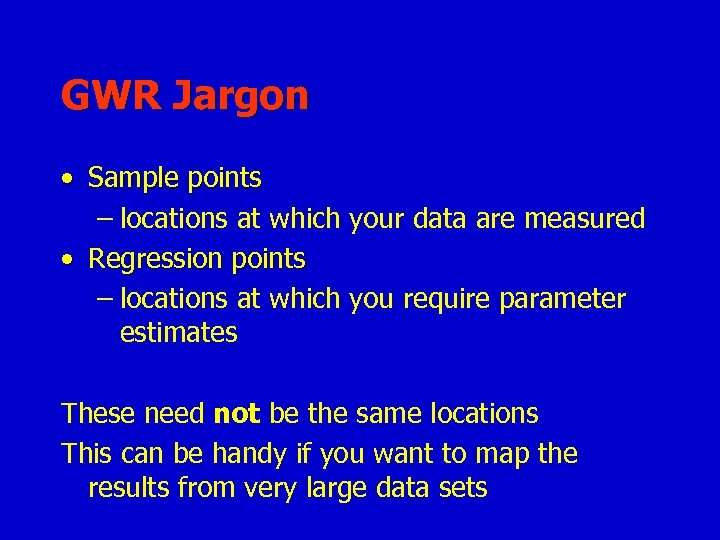GWR Jargon • Sample points – locations at which your data are measured • Regression points – locations at which you require parameter estimates These need not be the same locations This can be handy if you want to map the results from very large data sets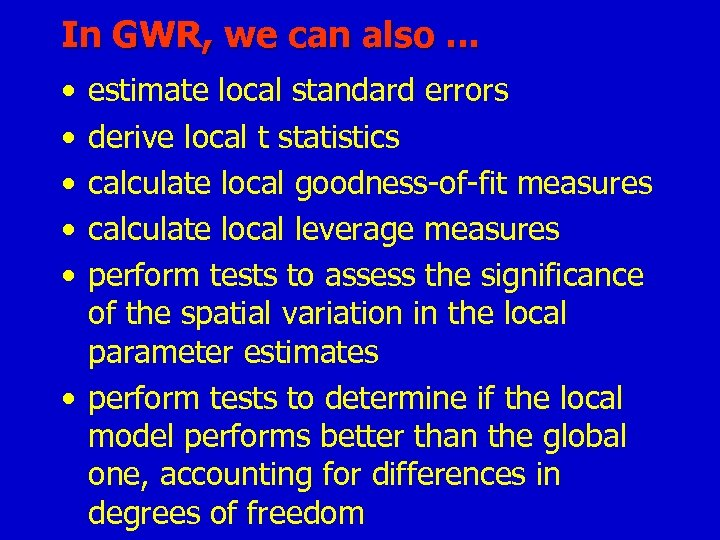In GWR, we can also. . . • • • estimate local standard errors derive local t statistics calculate local goodness-of-fit measures calculate local leverage measures perform tests to assess the significance of the spatial variation in the local parameter estimates • perform tests to determine if the local model performs better than the global one, accounting for differences in degrees of freedom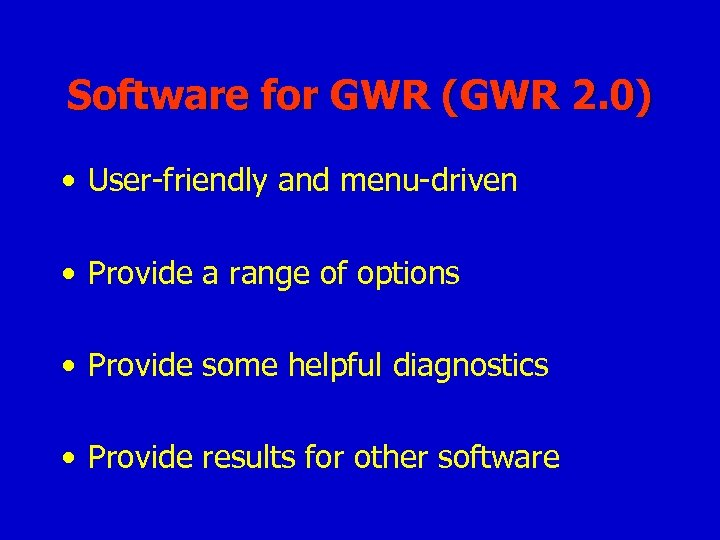Software for GWR (GWR 2. 0) • User-friendly and menu-driven • Provide a range of options • Provide some helpful diagnostics • Provide results for other softwareThe architecture of GWR 2. 0 • Currently about 3000 lines of FORTRAN • VB front-end to create a control file and run the program • Can run FORTRAN code under Unix with a control file or, more easily, by using the VB model editor in Windows (95; 98; ME)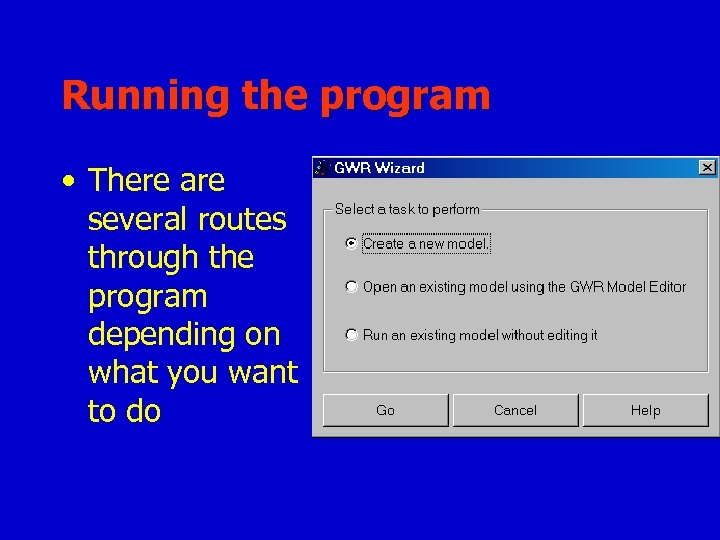Running the program • There are several routes through the program depending on what you want to do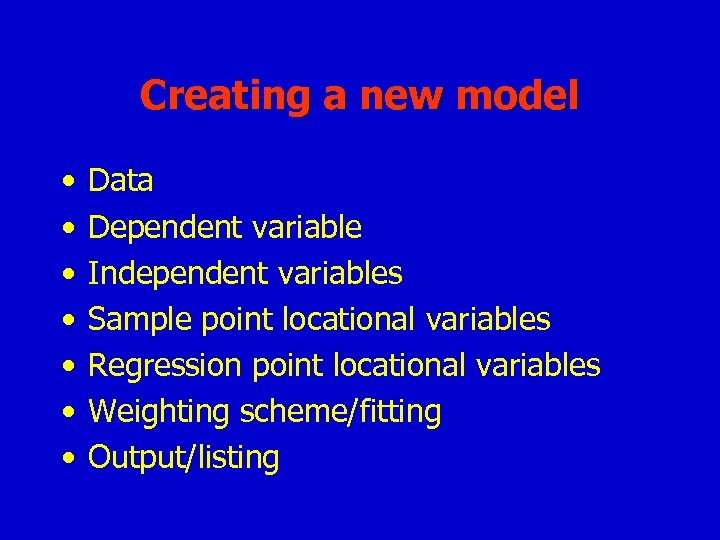Creating a new model • • Data Dependent variable Independent variables Sample point locational variables Regression point locational variables Weighting scheme/fitting Output/listing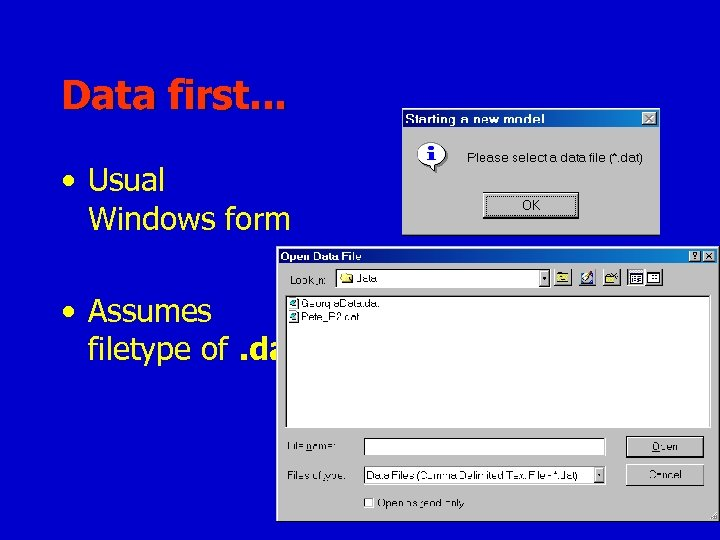Data first. . . • Usual Windows form • Assumes filetype of. dat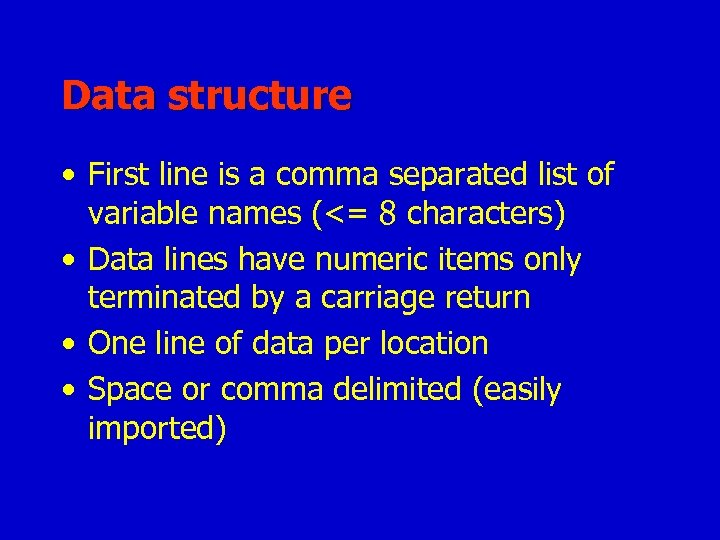Data structure • First line is a comma separated list of variable names (<= 8 characters) • Data lines have numeric items only terminated by a carriage return • One line of data per location • Space or comma delimited (easily imported)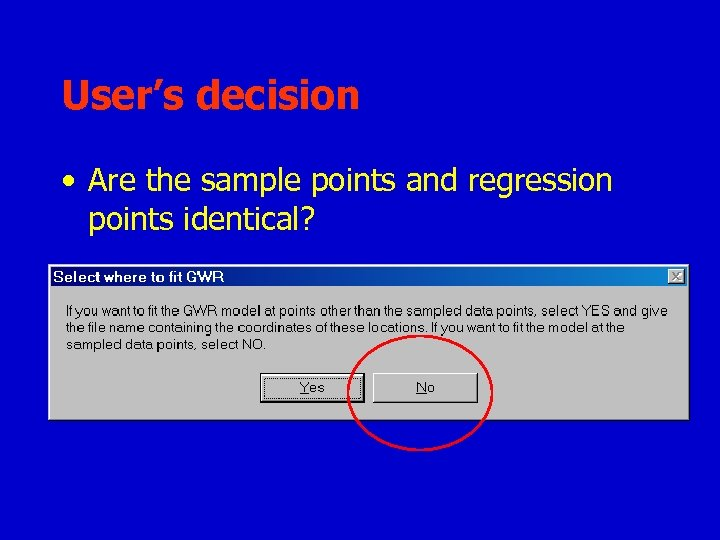User’s decision • Are the sample points and regression points identical?Output File • This will appear in the results folder • What type do you want?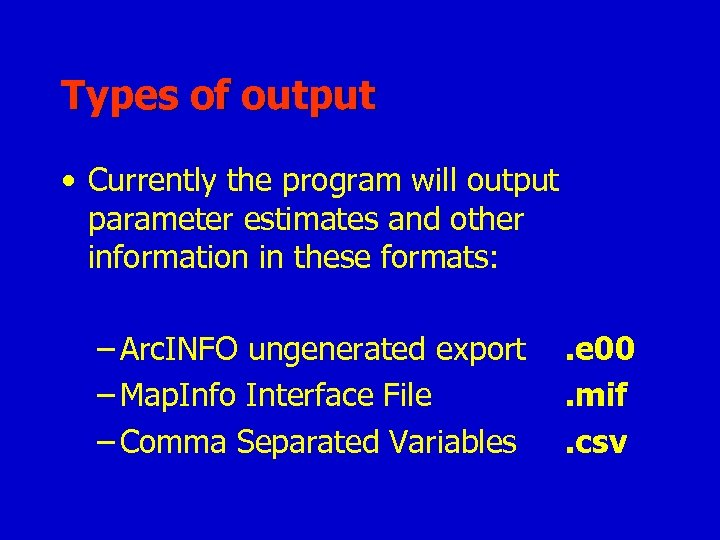Types of output • Currently the program will output parameter estimates and other information in these formats: – Arc. INFO ungenerated export – Map. Info Interface File – Comma Separated Variables . e 00. mif. csv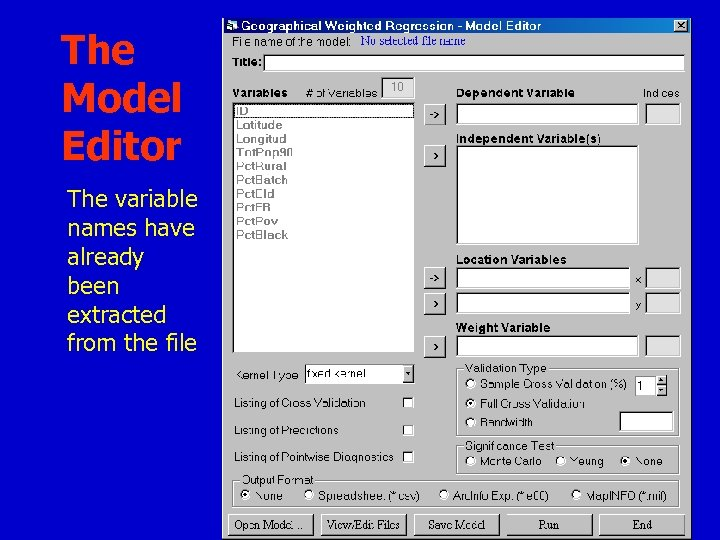The Model Editor The variable names have already been extracted from the file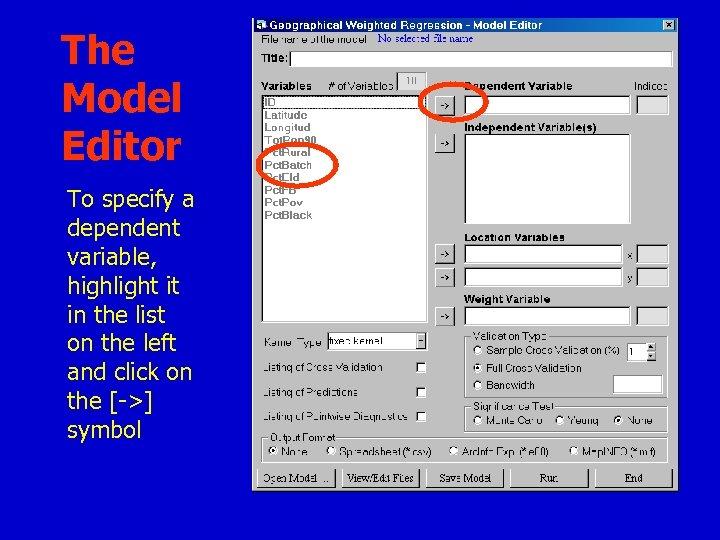The Model Editor To specify a dependent variable, highlight it in the list on the left and click on the [->] symbol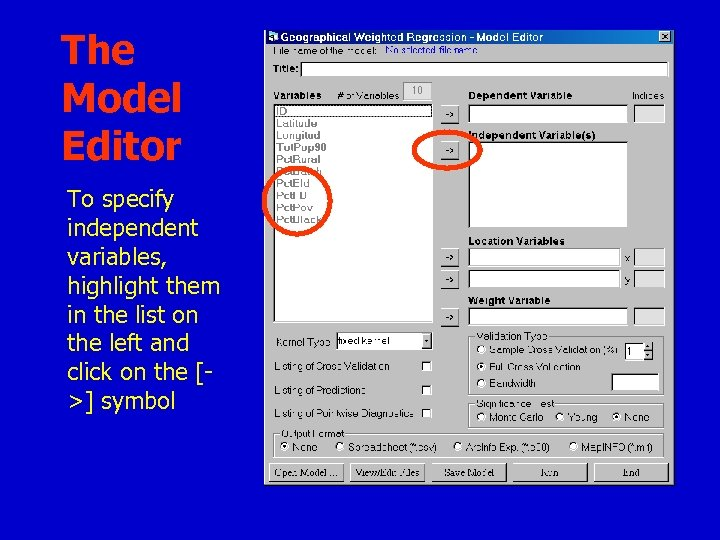The Model Editor To specify independent variables, highlight them in the list on the left and click on the [>] symbol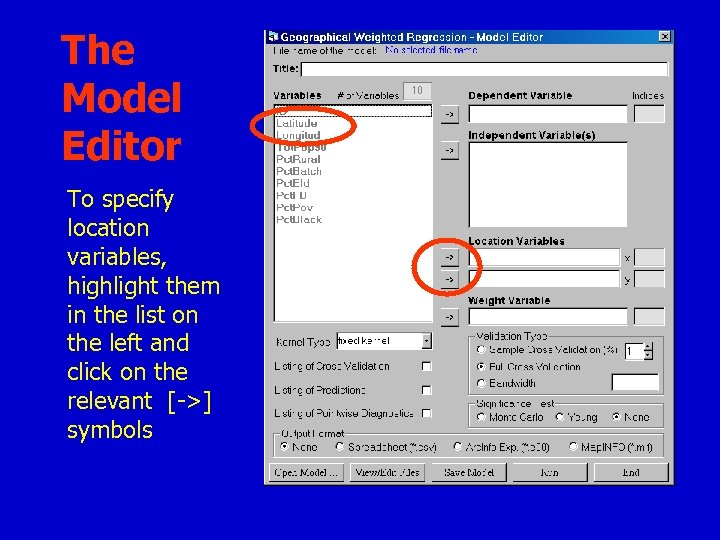The Model Editor To specify location variables, highlight them in the list on the left and click on the relevant [->] symbols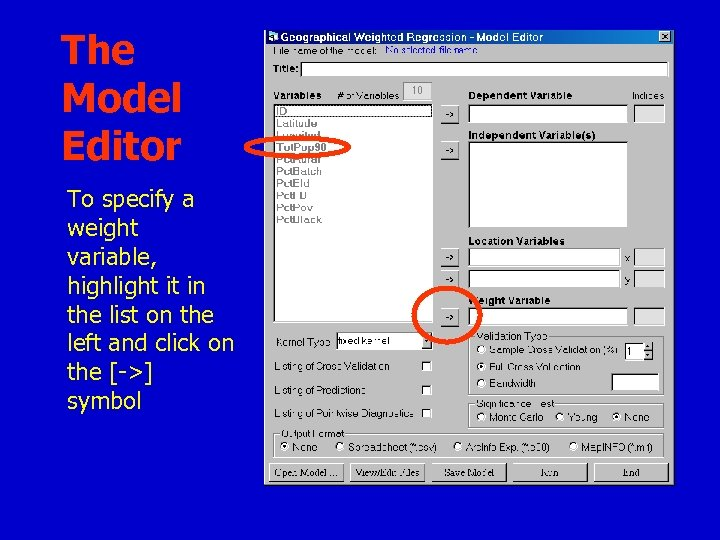The Model Editor To specify a weight variable, highlight it in the list on the left and click on the [->] symbol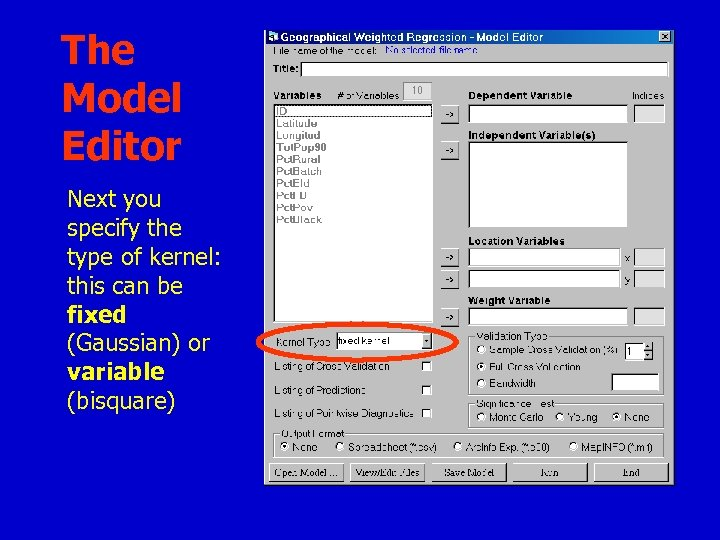The Model Editor Next you specify the type of kernel: this can be fixed (Gaussian) or variable (bisquare)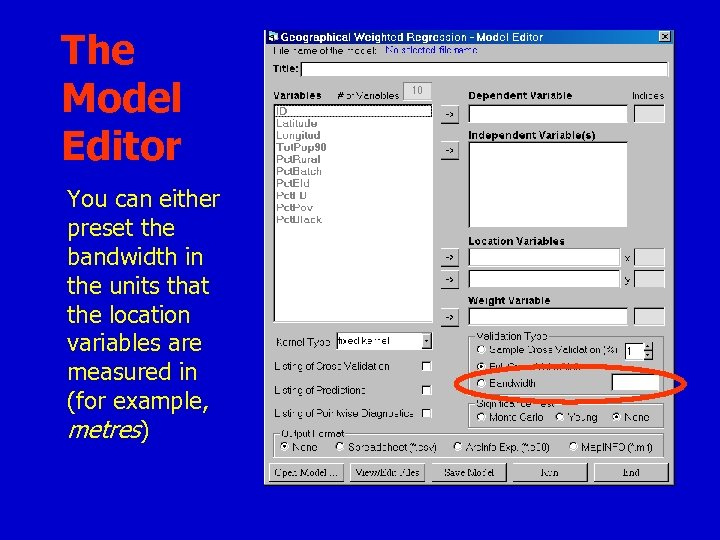The Model Editor You can either preset the bandwidth in the units that the location variables are measured in (for example, metres)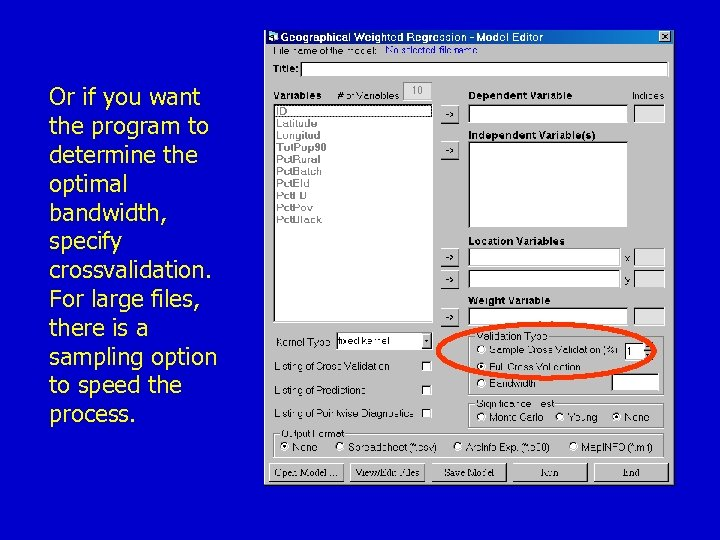Or if you want the program to determine the optimal bandwidth, specify crossvalidation. For large files, there is a sampling option to speed the process.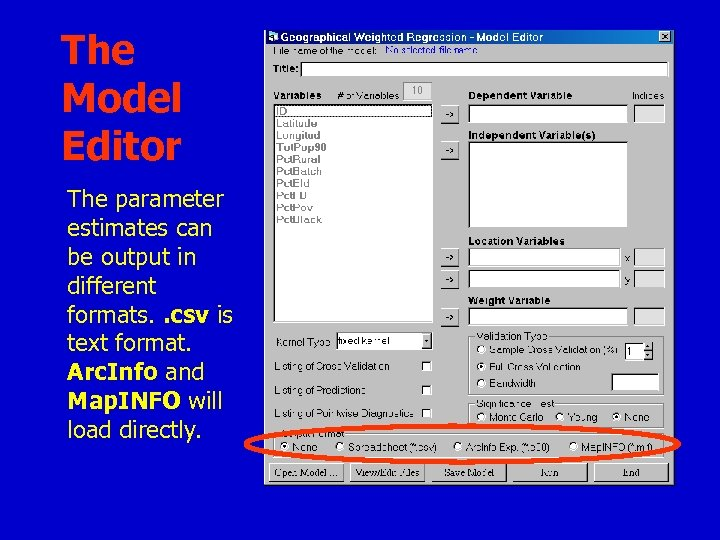The Model Editor The parameter estimates can be output in different formats. . csv is text format. Arc. Info and Map. INFO will load directly.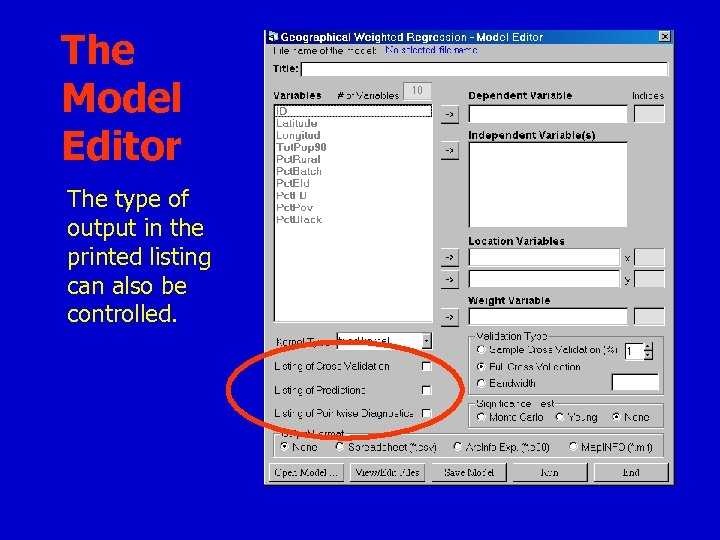The Model Editor The type of output in the printed listing can also be controlled.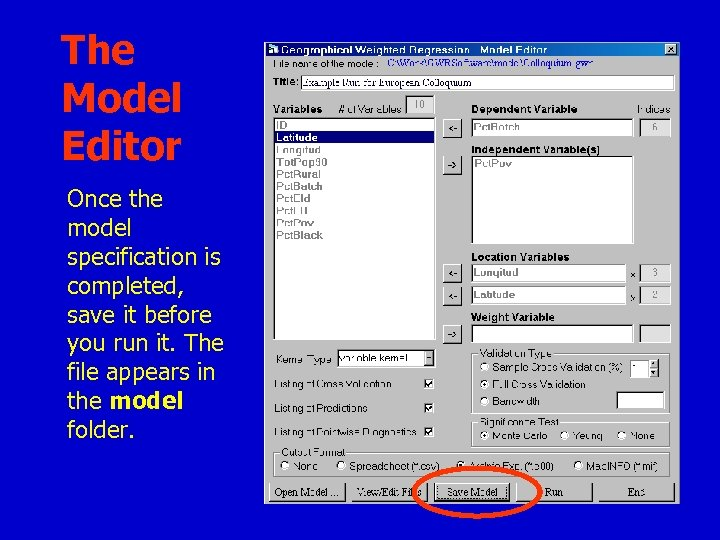The Model Editor Once the model specification is completed, save it before you run it. The file appears in the model folder.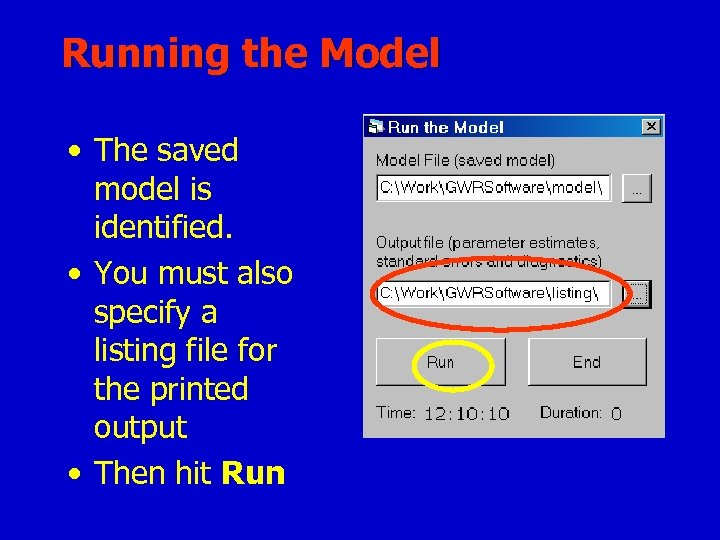Running the Model • The saved model is identified. • You must also specify a listing file for the printed output • Then hit Run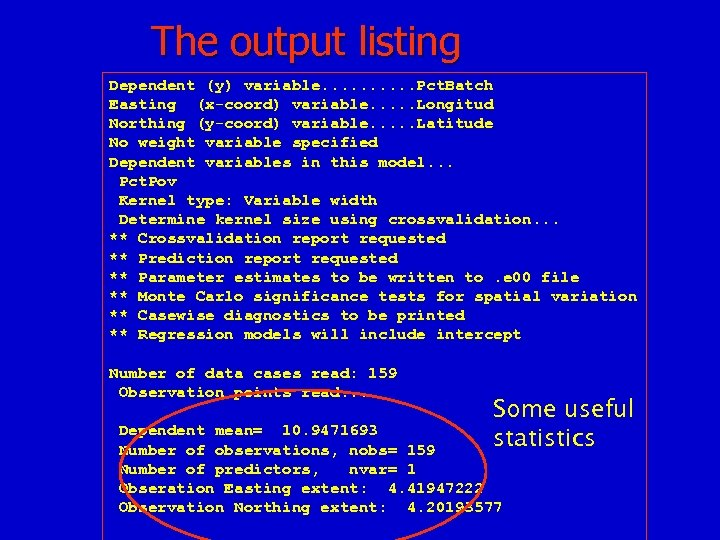The output listing Dependent (y) variable. . Pct. Batch Easting (x-coord) variable. . . Longitud Northing (y-coord) variable. . . Latitude No weight variable specified Dependent variables in this model. . . Pct. Pov Kernel type: Variable width Determine kernel size using crossvalidation. . . ** Crossvalidation report requested ** Prediction report requested ** Parameter estimates to be written to. e 00 file ** Monte Carlo significance tests for spatial variation ** Casewise diagnostics to be printed ** Regression models will include intercept Number of data cases read: 159 Observation points read. . . Some useful statistics Dependent mean= 10. 9471693 Number of observations, nobs= 159 Number of predictors, nvar= 1 Obseration Easting extent: 4. 41947222 Observation Northing extent: 4. 20193577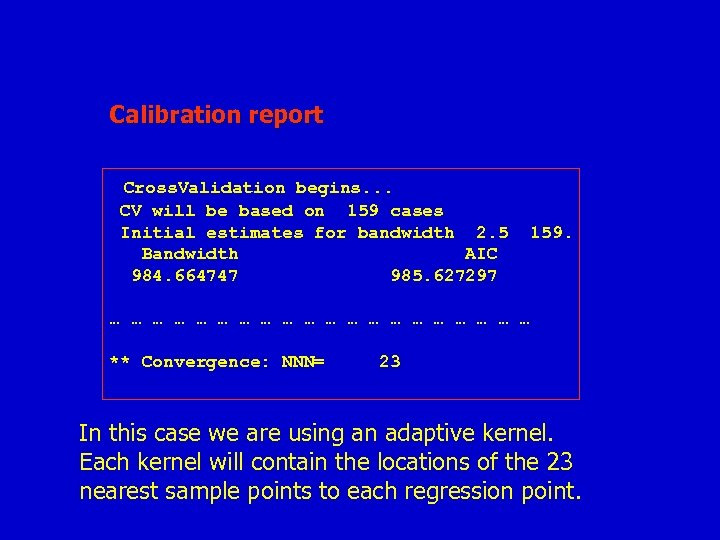Calibration report Cross. Validation begins. . . CV will be based on 159 cases Initial estimates for bandwidth 2. 5 Bandwidth AIC 984. 664747 985. 627297 159. … … … … … ** Convergence: NNN= 23 In this case we are using an adaptive kernel. Each kernel will contain the locations of the 23 nearest sample points to each regression point.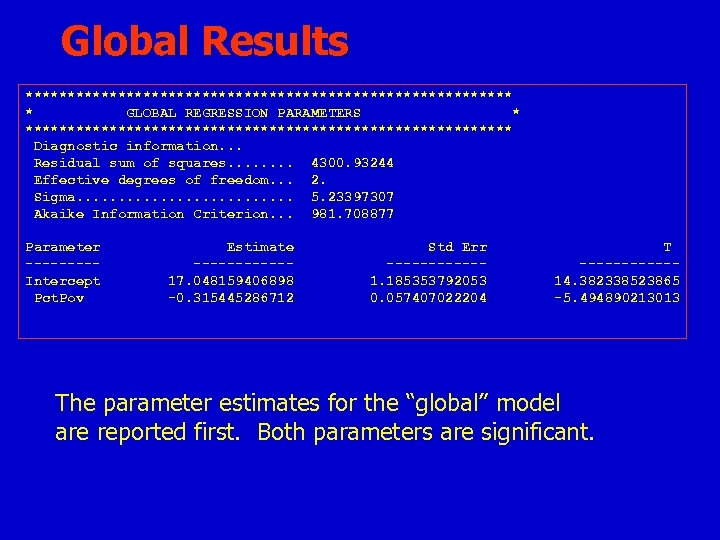Global Results ***************************** * GLOBAL REGRESSION PARAMETERS * ***************************** Diagnostic information. . . Residual sum of squares. . . . 4300. 93244 Effective degrees of freedom. . . 2. Sigma. . . 5. 23397307 Akaike Information Criterion. . . 981. 708877 Parameter ----Intercept Pct. Pov Estimate ------17. 048159406898 -0. 315445286712 Std Err ------1. 185353792053 0. 057407022204 T ------14. 382338523865 -5. 494890213013 The parameter estimates for the “global” model are reported first. Both parameters are significant.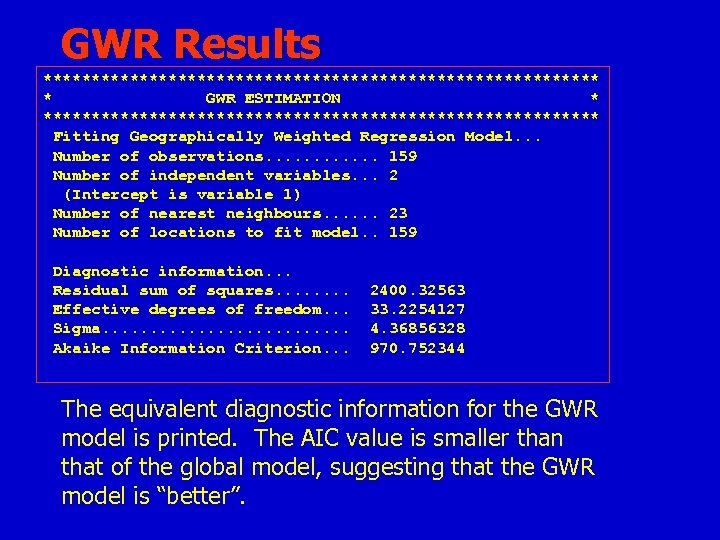GWR Results ***************************** * GWR ESTIMATION * ***************************** Fitting Geographically Weighted Regression Model. . . Number of observations. . . 159 Number of independent variables. . . 2 (Intercept is variable 1) Number of nearest neighbours. . . 23 Number of locations to fit model. . 159 Diagnostic information. . . Residual sum of squares. . . . Effective degrees of freedom. . . Sigma. . . Akaike Information Criterion. . . 2400. 32563 33. 2254127 4. 36856328 970. 752344 The equivalent diagnostic information for the GWR model is printed. The AIC value is smaller than that of the global model, suggesting that the GWR model is “better”.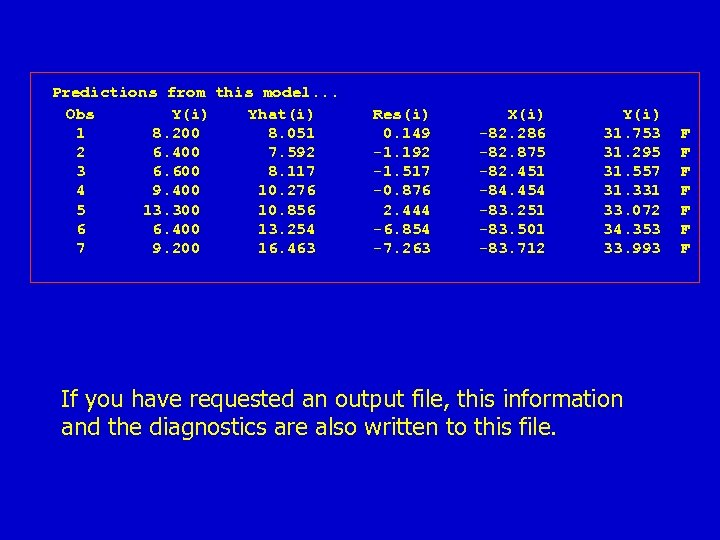Predictions from this model. . . Obs Y(i) Yhat(i) 1 8. 200 8. 051 2 6. 400 7. 592 3 6. 600 8. 117 4 9. 400 10. 276 5 13. 300 10. 856 6 6. 400 13. 254 7 9. 200 16. 463 Res(i) 0. 149 -1. 192 -1. 517 -0. 876 2. 444 -6. 854 -7. 263 X(i) -82. 286 -82. 875 -82. 451 -84. 454 -83. 251 -83. 501 -83. 712 Y(i) 31. 753 31. 295 31. 557 31. 331 33. 072 34. 353 33. 993 If you have requested an output file, this information and the diagnostics are also written to this file. F F F F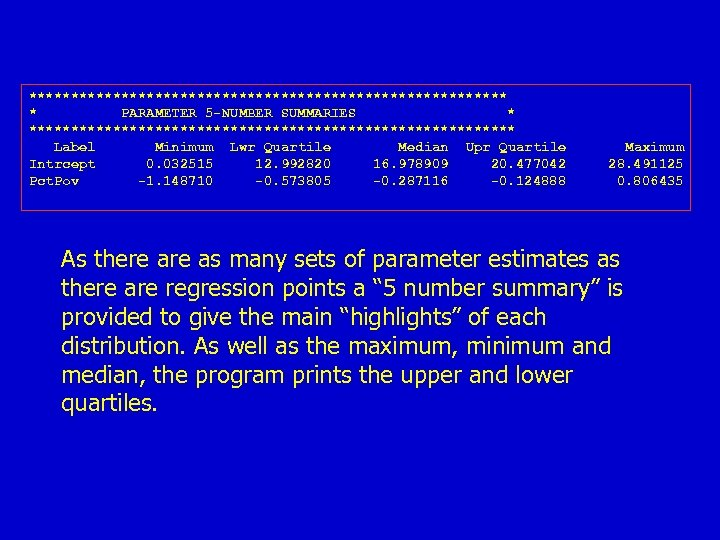***************************** * PARAMETER 5 -NUMBER SUMMARIES * ***************************** Label Minimum Lwr Quartile Median Upr Quartile Intrcept 0. 032515 12. 992820 16. 978909 20. 477042 Pct. Pov -1. 148710 -0. 573805 -0. 287116 -0. 124888 Maximum 28. 491125 0. 806435 As there as many sets of parameter estimates as there are regression points a “ 5 number summary” is provided to give the main “highlights” of each distribution. As well as the maximum, minimum and median, the program prints the upper and lower quartiles.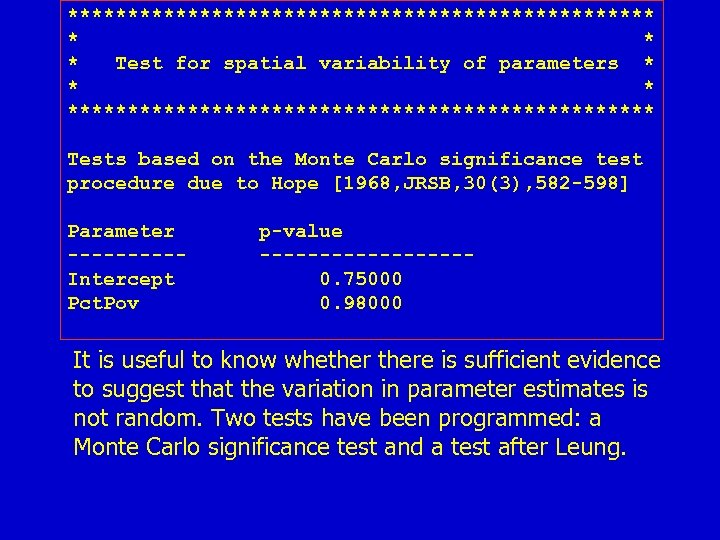************************* * Test for spatial variability of parameters * ************************* Tests based on the Monte Carlo significance test procedure due to Hope [1968, JRSB, 30(3), 582 -598] Parameter -----Intercept Pct. Pov p-value ---------0. 75000 0. 98000 It is useful to know whethere is sufficient evidence to suggest that the variation in parameter estimates is not random. Two tests have been programmed: a Monte Carlo significance test and a test after Leung.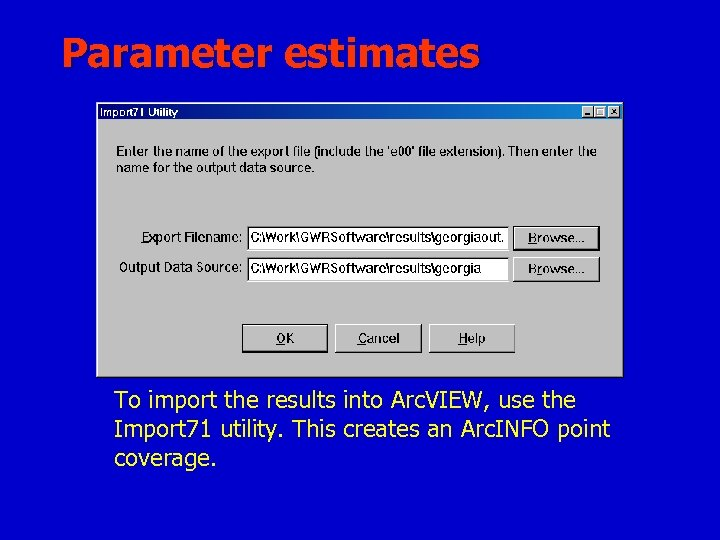Parameter estimates To import the results into Arc. VIEW, use the Import 71 utility. This creates an Arc. INFO point coverage.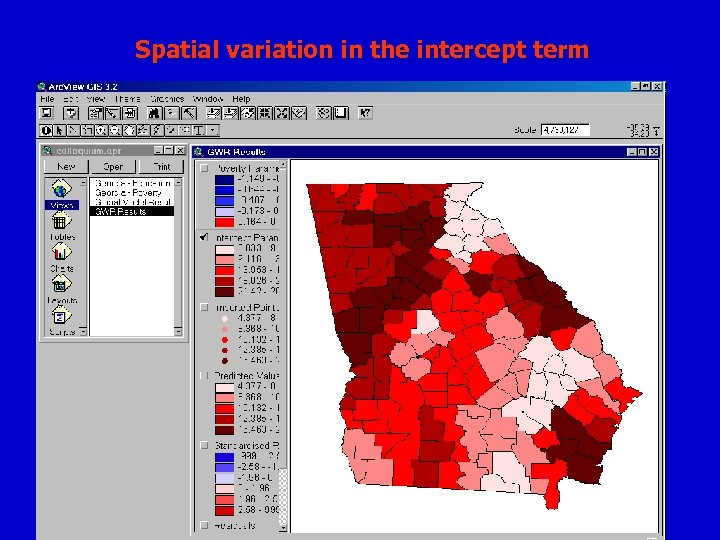Spatial variation in the intercept term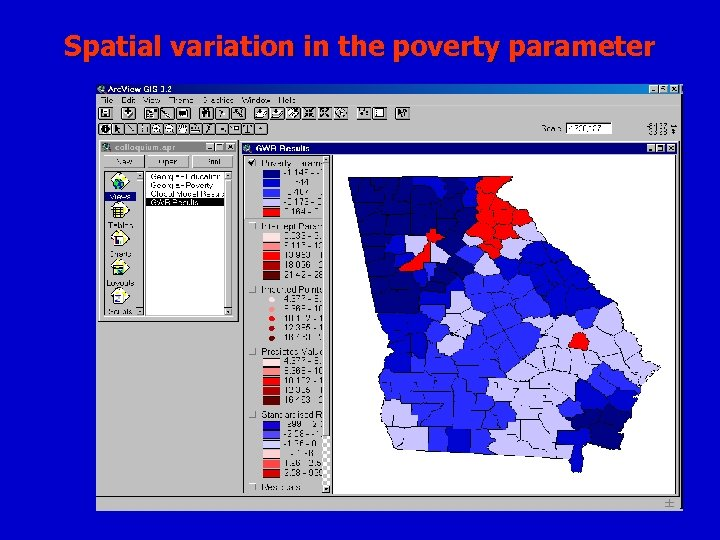Spatial variation in the poverty parameter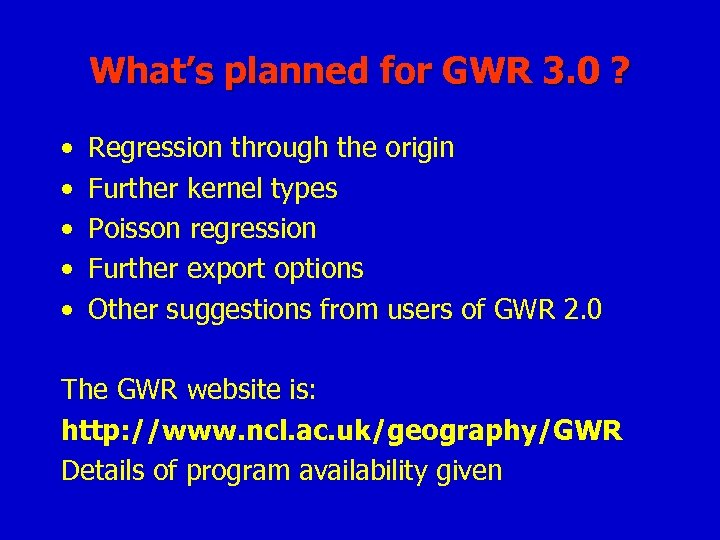What’s planned for GWR 3. 0 ? • • • Regression through the origin Further kernel types Poisson regression Further export options Other suggestions from users of GWR 2. 0 The GWR website is: http: //www. ncl. ac. uk/geography/GWR Details of program availability given